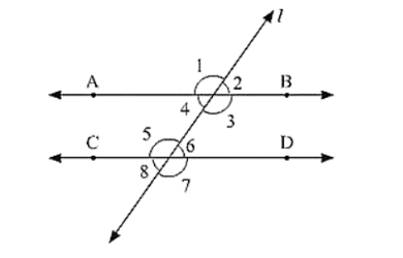# In below fig. AB CD and ∠1 and ∠2 are in the ratio 3: 2. Determine all angles from 1 to 8.

Question:

In below fig. AB CD and ∠1 and ∠2 are in the ratio 3: 2. Determine all angles from 1 to 8.Solution:

Let ∠1 = 3x and ∠2 = 2x

∠1 and ∠2 are linear pair of angle

Now, ∠1 and ∠2

⟹ 3x + 2x = 180

⟹ 5x = 180

⟹ x = 180/5

⟹ x = 36

∠1 = 3x = 108°,∠2 = 2x = 72°

We know, Vertically opposite angles are equal

∠1 = ∠3 = 108°

∠2 = ∠4 = 72°

∠6 = ∠7 = 108°

∠5 = ∠8 = 72°

We also know, corresponding angles are equal

∠1 = ∠5 = 108°

∠2 = ∠6 = 72°